5.4 Interference in Space: Patterns

In the previous section, we considered two geometries for the interference of two waves with the same frequency. In the first case, the waves were traveling in the same direction and in the second, the waves were traveling at each other in opposite directions. In both case, we considered whether we would encounter constructive or destructive interference as we moved along the same line that the waves were moving. This reduced the problem to one dimension, just as we began our discussion of waves in Chapter 3. Although one dimension is easier to understand, many important effects happen in two or three dimensions, so in this section, we will consider a two dimensional geometry. Under these conditions, the points of constructive and destructive interference form a complex pattern, which we will refer to as an interference pattern.

To begin the discussion, consider two sources of sound that give off waves in all directions. One source and wave is colored red, while the other is green. Initially, the sources are right on top of each other, so you cannot see the red source: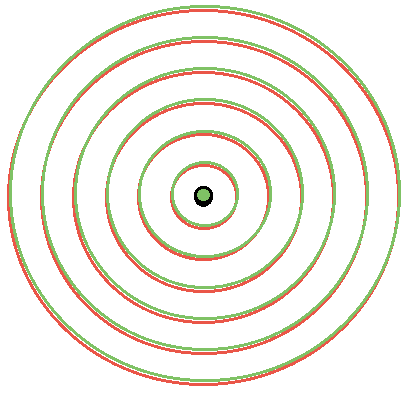Since the sources are right on top of each other, wherever you stand, you will be equidistant from each source. Thus, the path difference to each source will be zero and there will be constructive interference everywhere.

Now, lets consider what happens if we move one speaker by half a wavelength in one direction: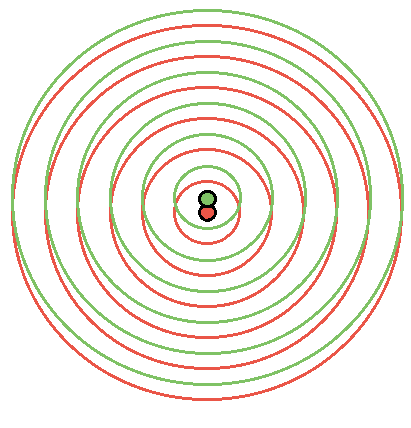Things begin to look more complicated. If we look along the horizontal (direction A), we find that no matter where we are on that line, we are always equidistant from each speaker. Therefore, we will find constructive interference. So, even though we moved one of the speakers, we still have constructive interference along A. This is not true for the vertical (direction B). Along this line, the speakers are displaced from each other by half a wavelength. In the last section, we found that under these conditions, the waves will interfere destructively, and we will not hear any sound in this direction. We can imagine ourselves walking in a big circle around the two speakers and marking the points where we find constructive (x) and destructive (· ) interference: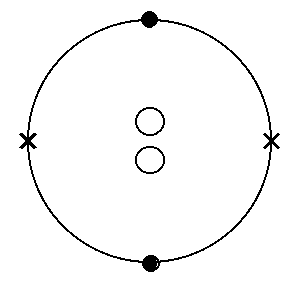Of course, we can keep going. Now, lets move the speakers apart by a full wavelength: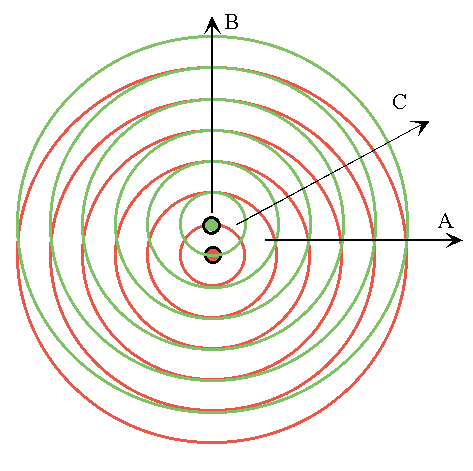The question is the same as before: where will we find constructive and destructive interference?Again, nothing has changed in direction A. We will always be equidistant from each speaker and get constructive interference. Along direction B, the speakers are now displaced by a full wavelength also giving constructive interference. So along the direction that the speaker is displaced, we go repeatedly through constructive and destructive interference. Now, in the present case, we have constructive interference at both A and B. Does this mean that we will have constructive interference at all points in between A and B? The answer is definitely NO. At A, the path difference is zero while at B the path difference is a wavelength, or l . As we move from A to B the path difference will change continuously from zero to l , so there must be some point where the path difference is exactly l /2. At this point, we will have destructive interference. This is marked in the figure above by C. The interference pattern along a circle surrounding the speakers now looks like this: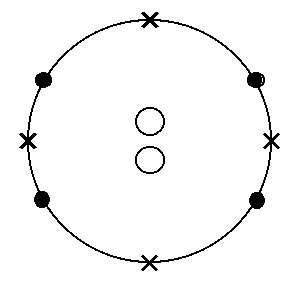And, of course, we can keep moving the speakers apart. Every time we move a half of a wavelength, the point at the top of the figure will go from constructive to destructive interference and a new point of constructive interference will appear on the circle, OR the point at the top of the figure will go from destructive to constructive interference and a new point of destructive interference will appear on the circle.

As the separation increases, the pattern will become increasingly complicated, but in principle we can figure out the pattern of constructive and destructive interference, if we know the separation of the two sources. To get a better idea of what these patterns look like, try out the Physlet entitled Interference.

Although the interference pattern can be quite complicated, there is one fairly simple aspect to it and which is also the most useful. So far, we considered the interference pattern as though we were walking in a big circle around the two sources of waves. Now let’s restrict our attention to a smaller region where we are pretty much right in front of the two sources: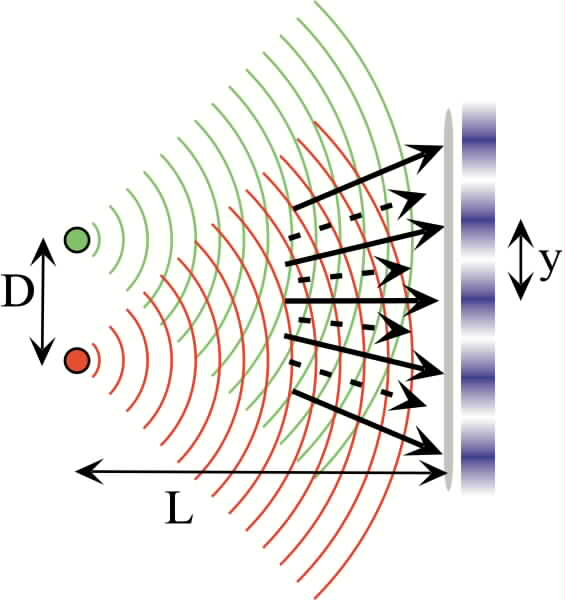In this picture, there are two sources of waves (red and green) separated by a distance D. If you look a distance L away from the two sources, you will find points of constructive and destructive interference (shown by the pattern in blue). The solid arrows show where there is constructive interference, while the dashed arrows show destructive interference. In turns out that there is a rather simple expression giving the spacing of the points of constructive interference: y = l D/L, where l is the wavelength of the wave. The derivation is given as an addition to this section, but is not important for this course.

This effect is important for many reasons. This particular interference pattern is fairly simple to setup and observe. One just places two sources close together and moves back a distance L. If the wavelength is known, one can measure the separation D. If the wavelength is very small, one can measure very small separations this way. Alternatively, if the separation is known, one can make a measurement of the wavelength of a wave.

However, even more important than these applications is the fact that if you do see an interference pattern, then you know that you are dealing with waves. How is this important? Consider the following photograph: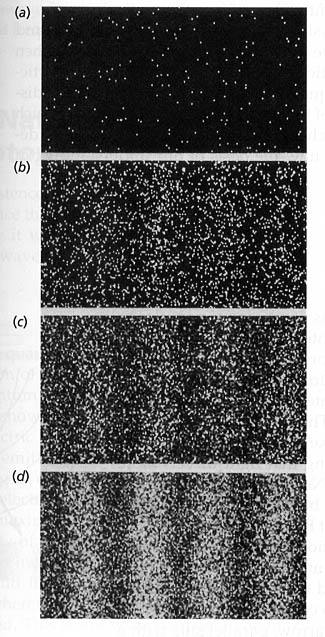The exposure is longer and longer going from (a) to (d). In (d) it is quite clear that there is an interference pattern. What makes this result so remarkable is that the source in this case was a beam of electrons! Now, electrons are particles that have a mass, like a rock or a baseball. Until the early 1900’s no one ever imagined that the matter that we are made of could be a wave. Many types of waves were understood, such as sound and light waves as well as the phenomenon of interference. So, when an interference pattern was made with electrons it proved that electrons were actually waves. This completely changed the course of physics and led to quantum mechanics and much of the modern technology that we take for granted.

One question is worth asking: why did it take so long to realize the wave nature of matter? We can get some idea by looking at the expression for the spacing of the bands in the interference pattern: y = l D/L. It just so happens that the wavelength of the waves that matter is composed of is very small. This makes the spacing, y, very small and, thus, hard to see. The interference pattern is very fine and easily blurred out. If you cannot make out the constructive and destructive interference, then you would not think that you are observing a wave. So, it took physicists some time to figure out how to actually see the interference pattern. You can also see this in the interference Physlet. If you make the wavelength very small, the pattern starts looking very strange. This is because the resolution of the screen that you are looking at is not good enough to accurately show the interference pattern properly.

The wavelength of sound waves in much greater than the wavelength of matter waves, so the interference of sound is much easier to observe, and indeed you will hear this clearly in one of the labs. As sound bounces around a room, it forms a very complex interference pattern that is hard to predict, but can lead to spots in the room that are loud due to constructive interference and spots that are soft due to destructive interference. Moreover, each frequency of sound has a different wavelength and so each frequency will have a different interference pattern making the sound characteristics change significantly from point to point. If you have ever watched an accomplished musician play, they often seem to sway, moving their heads quite a bit. It is thought that this is an instinctive response to the complex interference pattern: by moving ones head, one can "average out" this interference pattern leading to a more accurate perception of the sound being produced.